# Sequence analysis How to locate rareimportant subsequences Sequence

• Slides: 69
Download presentationSequence analysis How to locate rare/important subsequences.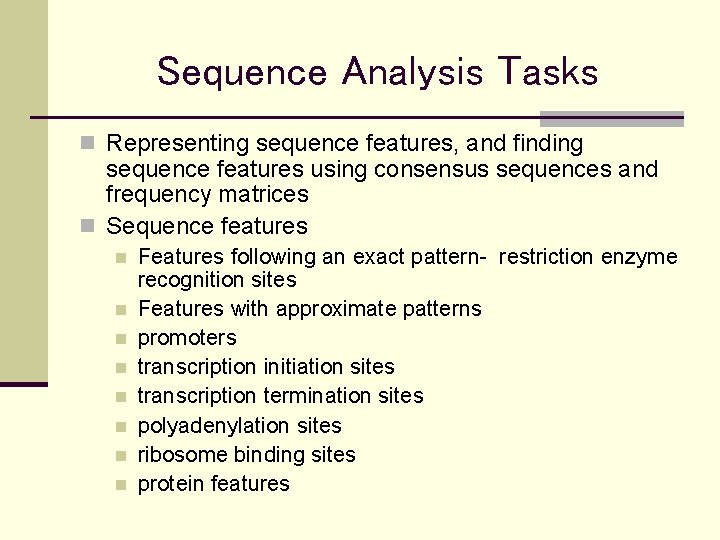Sequence Analysis Tasks n Representing sequence features, and finding sequence features using consensus sequences and frequency matrices n Sequence features n n n n Features following an exact pattern- restriction enzyme recognition sites Features with approximate patterns promoters transcription initiation sites transcription termination sites polyadenylation sites ribosome binding sites protein features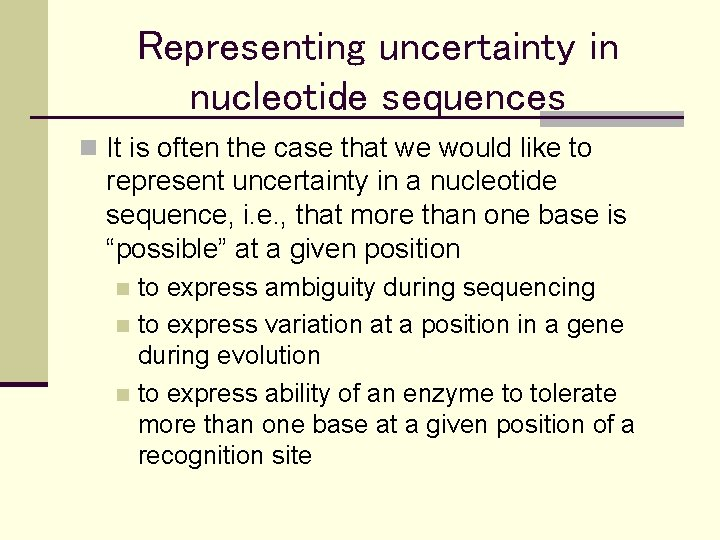Representing uncertainty in nucleotide sequences n It is often the case that we would like to represent uncertainty in a nucleotide sequence, i. e. , that more than one base is “possible” at a given position to express ambiguity during sequencing n to express variation at a position in a gene during evolution n to express ability of an enzyme to tolerate more than one base at a given position of a recognition site n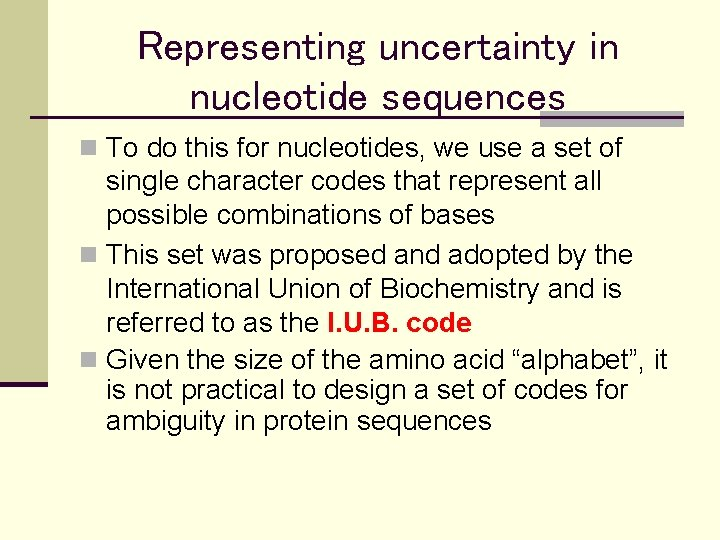Representing uncertainty in nucleotide sequences n To do this for nucleotides, we use a set of single character codes that represent all possible combinations of bases n This set was proposed and adopted by the International Union of Biochemistry and is referred to as the I. U. B. code n Given the size of the amino acid “alphabet”, it is not practical to design a set of codes for ambiguity in protein sequences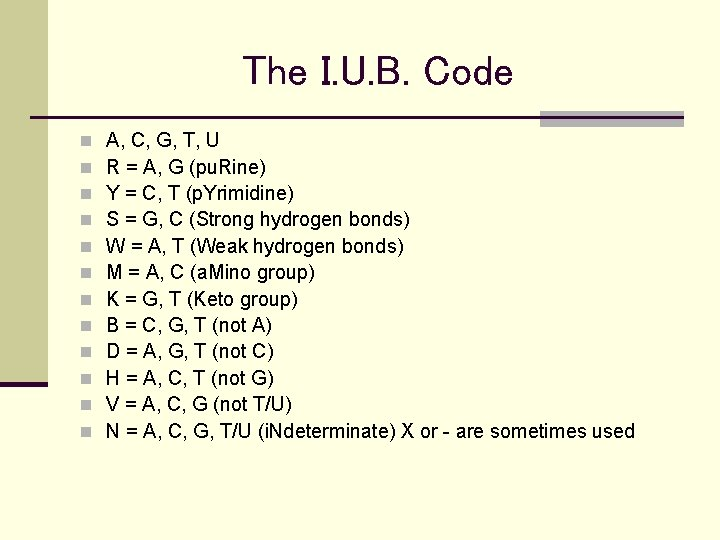The I. U. B. Code n n n A, C, G, T, U R = A, G (pu. Rine) Y = C, T (p. Yrimidine) S = G, C (Strong hydrogen bonds) W = A, T (Weak hydrogen bonds) M = A, C (a. Mino group) K = G, T (Keto group) B = C, G, T (not A) D = A, G, T (not C) H = A, C, T (not G) V = A, C, G (not T/U) N = A, C, G, T/U (i. Ndeterminate) X or - are sometimes used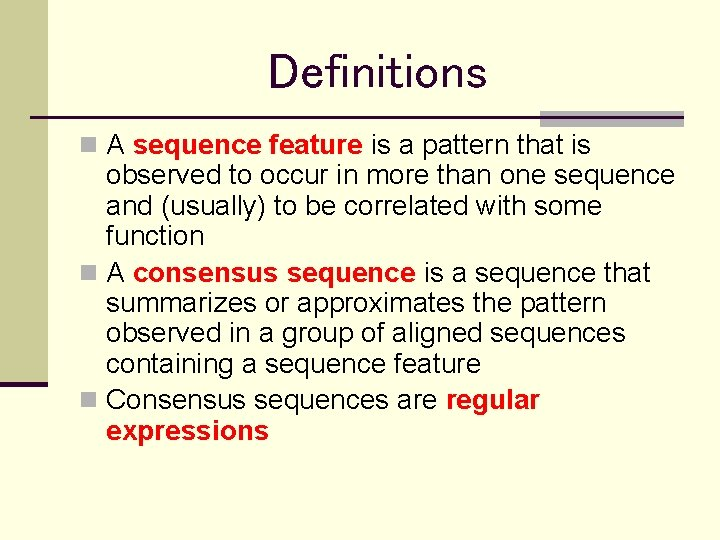Definitions n A sequence feature is a pattern that is observed to occur in more than one sequence and (usually) to be correlated with some function n A consensus sequence is a sequence that summarizes or approximates the pattern observed in a group of aligned sequences containing a sequence feature n Consensus sequences are regular expressions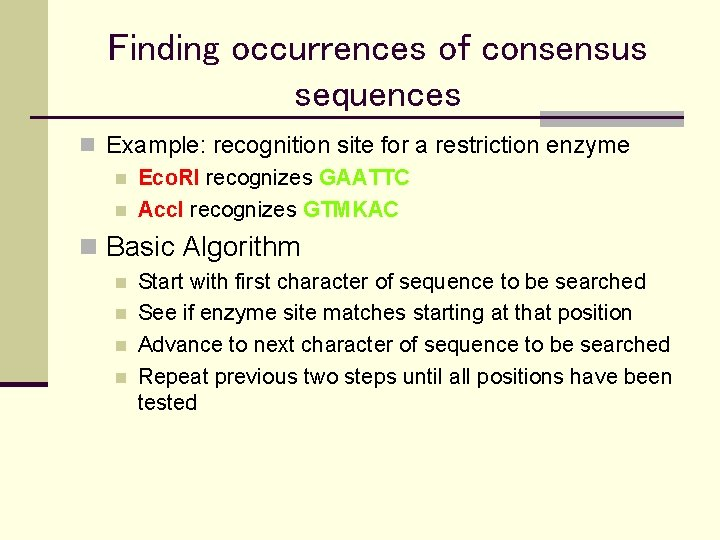Finding occurrences of consensus sequences n Example: recognition site for a restriction enzyme n Eco. RI recognizes GAATTC n Acc. I recognizes GTMKAC n Basic Algorithm n n Start with first character of sequence to be searched See if enzyme site matches starting at that position Advance to next character of sequence to be searched Repeat previous two steps until all positions have been tested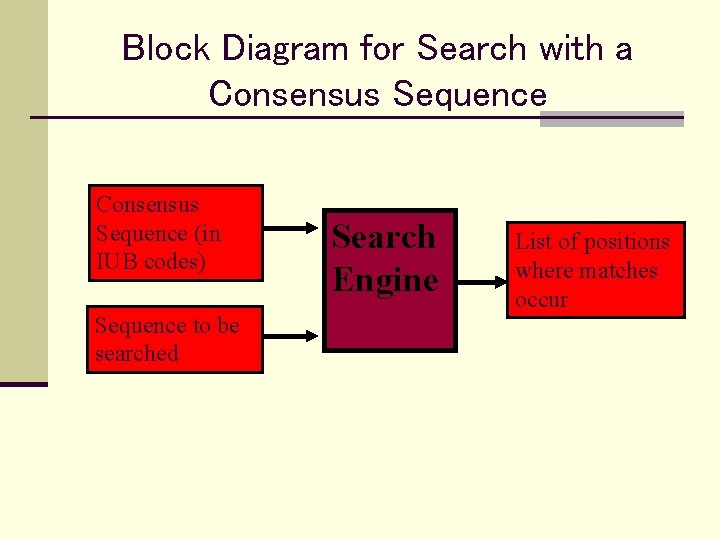Block Diagram for Search with a Consensus Sequence (in IUB codes) Sequence to be searched Search Engine List of positions where matches occurStatistics of pattern appearance n Goal: Determine the significance of observing a feature (pattern) n Method: Estimate the probability that pattern would occur randomly in a given sequence. Three different methods n n n Assume all nucleotides are equally frequent Use measured frequencies of each nucleotide (mononucleotide frequencies) Use measured frequencies with which a given nucleotide follows another (dinucleotide frequencies)Determining mononucleotide frequencies n Count how many times each nucleotide appears in n n sequence Divide (normalize) by total number of nucleotides Result: f. A mononucleotide frequency of A (frequency that A is observed) Define: p. A mononucleotide probability that a nucleotide will be an A p. A assumed to equal f. A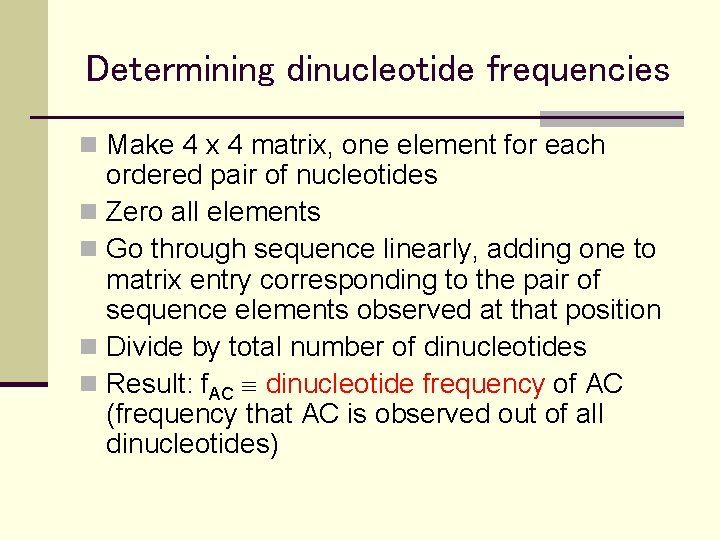Determining dinucleotide frequencies n Make 4 x 4 matrix, one element for each ordered pair of nucleotides n Zero all elements n Go through sequence linearly, adding one to matrix entry corresponding to the pair of sequence elements observed at that position n Divide by total number of dinucleotides n Result: f. AC dinucleotide frequency of AC (frequency that AC is observed out of all dinucleotides)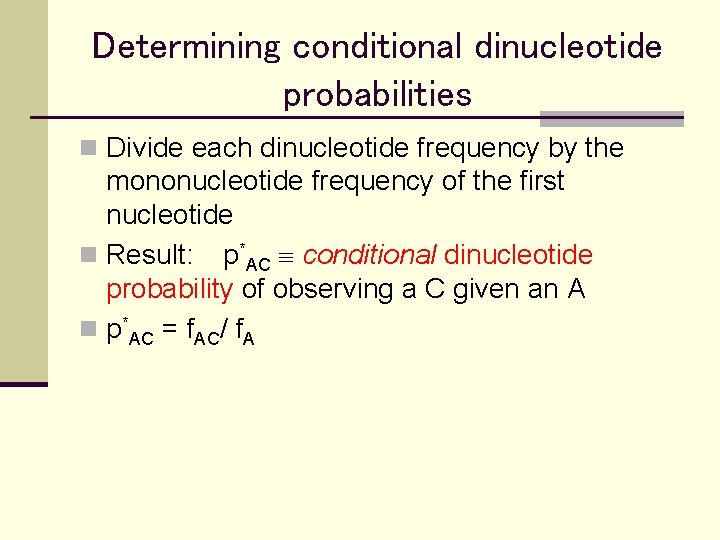Determining conditional dinucleotide probabilities n Divide each dinucleotide frequency by the mononucleotide frequency of the first nucleotide n Result: p*AC conditional dinucleotide probability of observing a C given an A n p*AC = f. AC/ f. A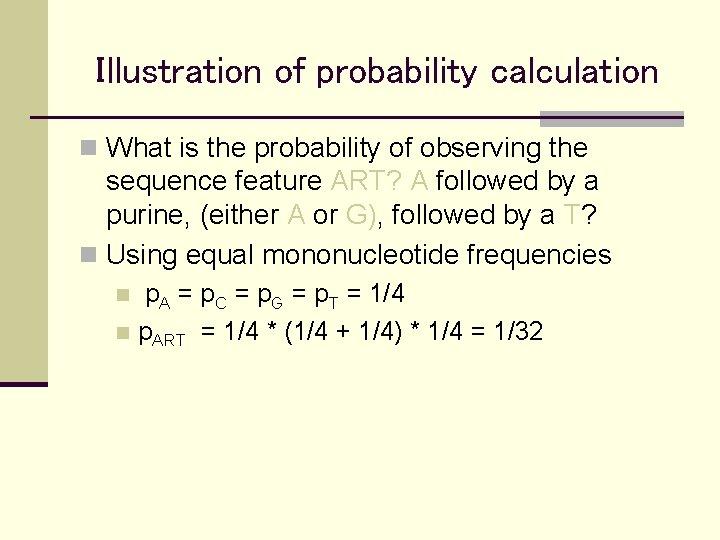Illustration of probability calculation n What is the probability of observing the sequence feature ART? A followed by a purine, (either A or G), followed by a T? n Using equal mononucleotide frequencies p. A = p. C = p. G = p. T = 1/4 n p. ART = 1/4 * (1/4 + 1/4) * 1/4 = 1/32 nIllustration (continued) n Using observed mononucleotide frequencies: n p. ART = p. A (p. A + p. G) p. T n Using dinucleotide frequencies: n p. ART = p. A (p*AAp*AT + p*AGp*GT)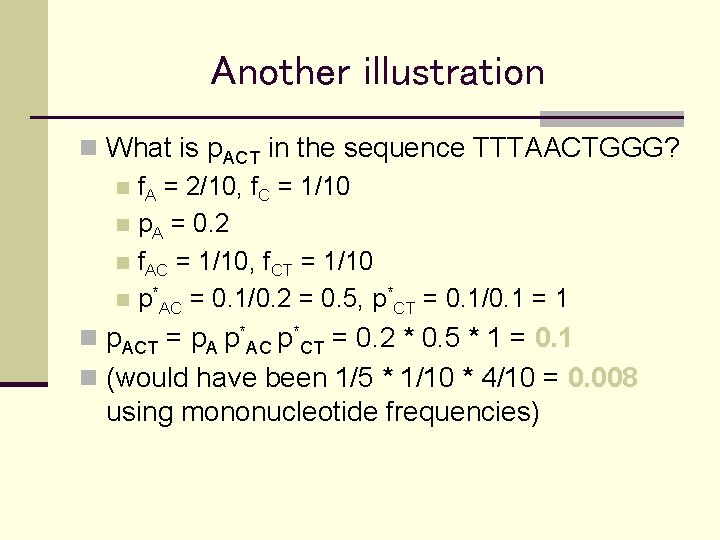Another illustration n What is p. ACT in the sequence TTTAACTGGG? n f. A = 2/10, f. C = 1/10 n p. A = 0. 2 n f. AC = 1/10, f. CT = 1/10 n p*AC = 0. 1/0. 2 = 0. 5, p*CT = 0. 1/0. 1 = 1 n p. ACT = p. A p*AC p*CT = 0. 2 * 0. 5 * 1 = 0. 1 n (would have been 1/5 * 1/10 * 4/10 = 0. 008 using mononucleotide frequencies)Expected number and spacing n Probabilities are per nucleotide n How do we calculate number of expected features in a sequence of length L? n Expected number (for large L) Lp n How do we calculate the expected spacing between features? n ART expected spacing between ART features = 1/p. ART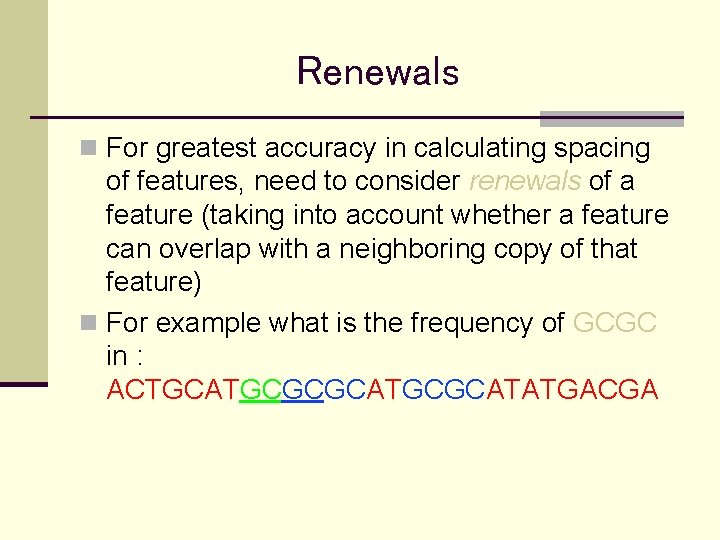Renewals n For greatest accuracy in calculating spacing of features, need to consider renewals of a feature (taking into account whether a feature can overlap with a neighboring copy of that feature) n For example what is the frequency of GCGC in : ACTGCATGCGCATATGACGARenewals n We define a renewal as the end of a non overlapping motif. n For example: The renewals of GCGC in ACTGCATGCGCATATGCGCGC GC Are at 11, 19, 27, 31 The clamps size are: 2, 1, 2, 1Renewals and Clump size. n Let R be a general pattern: R=(r 1, …, rm) n Let us denote: R(i)=(r 1, …, ri) R(i)=(rm-i+1, …, rm) n The clamp size is:Clamp Frequency n Let us assume that the clamps are distributed randomly. Their frequency, and the interval between any two clamps would be: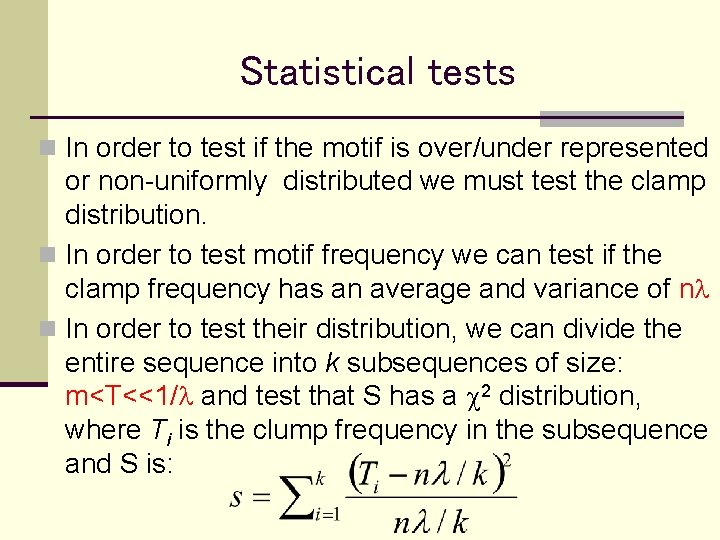Statistical tests n In order to test if the motif is over/under represented or non-uniformly distributed we must test the clamp distribution. n In order to test motif frequency we can test if the clamp frequency has an average and variance of nl n In order to test their distribution, we can divide the entire sequence into k subsequences of size: m<T<<1/l and test that S has a c 2 distribution, where Ti is the clump frequency in the subsequence and S is:Frequency of simple motifsStatistics of AT- or GC-rich regions n What is the probability of observing a “run” of the same nucleotide (e. g. , 25 A’s) n Let px be the mononucleotide probability of nucleotide x n The per nucleotide probability of a run of N consecutive x’s is px. N n The probability of occurrence in a sequence of length L much longer than N is ≈ L px. N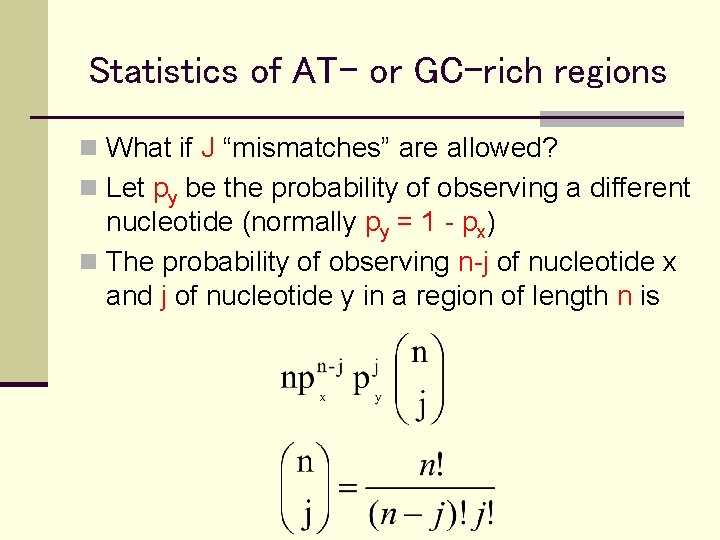Statistics of AT- or GC-rich regions n What if J “mismatches” are allowed? n Let py be the probability of observing a different nucleotide (normally py = 1 - px) n The probability of observing n-j of nucleotide x and j of nucleotide y in a region of length n is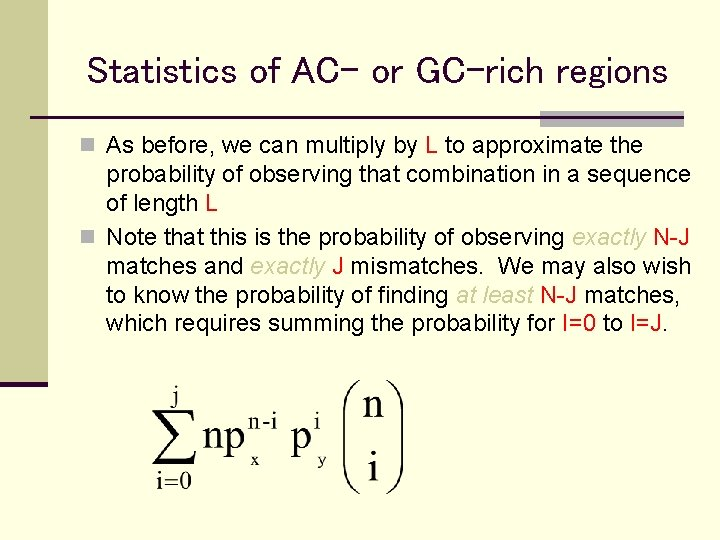Statistics of AC- or GC-rich regions n As before, we can multiply by L to approximate the probability of observing that combination in a sequence of length L n Note that this is the probability of observing exactly N-J matches and exactly J mismatches. We may also wish to know the probability of finding at least N-J matches, which requires summing the probability for I=0 to I=J.Frequency matrices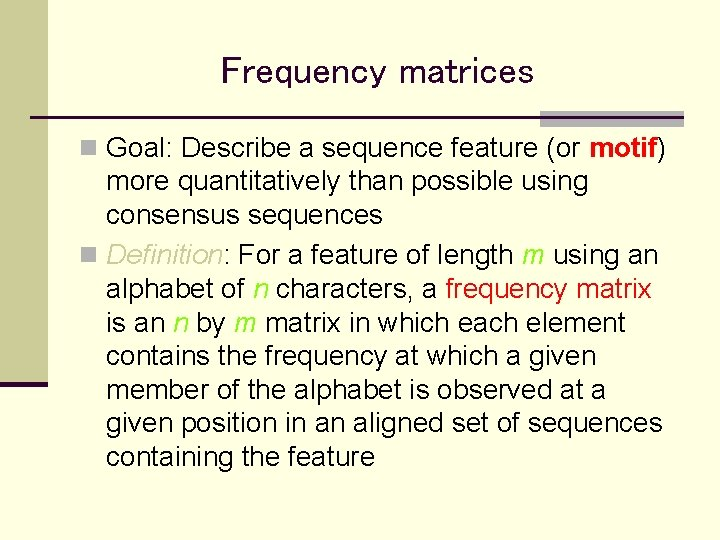Frequency matrices n Goal: Describe a sequence feature (or motif) more quantitatively than possible using consensus sequences n Definition: For a feature of length m using an alphabet of n characters, a frequency matrix is an n by m matrix in which each element contains the frequency at which a given member of the alphabet is observed at a given position in an aligned set of sequences containing the feature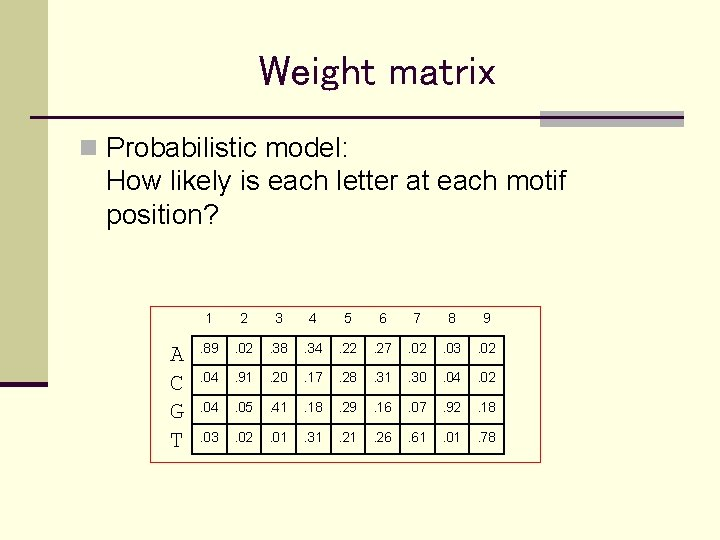Weight matrix n Probabilistic model: How likely is each letter at each motif position? A C G T 1 2 3 4 5 6 7 8 9 . 89 . 02 . 38 . 34 . 22 . 27 . 02 . 03 . 02 . 04 . 91 . 20 . 17 . 28 . 31 . 30 . 04 . 02 . 04 . 05 . 41 . 18 . 29 . 16 . 07 . 92 . 18 . 03 . 02 . 01 . 31 . 26 . 61 . 01 . 78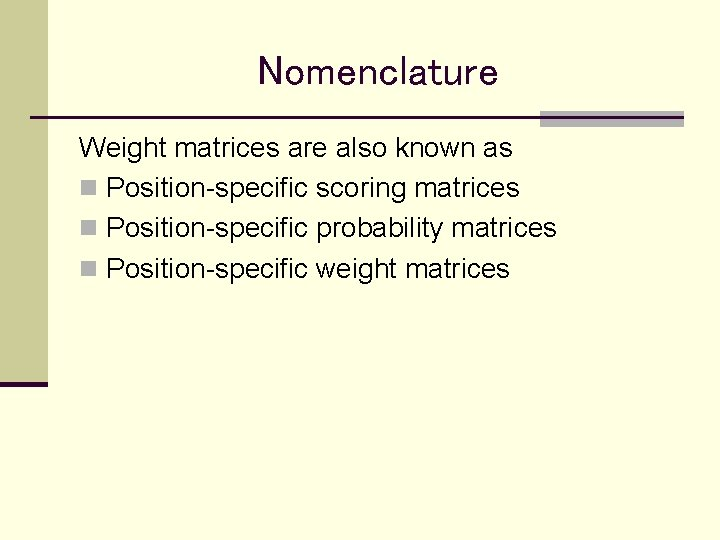Nomenclature Weight matrices are also known as n Position-specific scoring matrices n Position-specific probability matrices n Position-specific weight matrices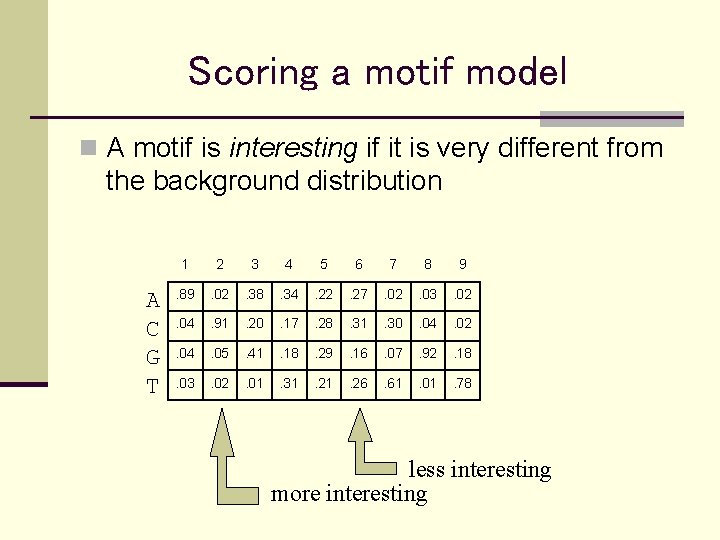Scoring a motif model n A motif is interesting if it is very different from the background distribution A C G T 1 2 3 4 5 6 7 8 9 . 89 . 02 . 38 . 34 . 22 . 27 . 02 . 03 . 02 . 04 . 91 . 20 . 17 . 28 . 31 . 30 . 04 . 02 . 04 . 05 . 41 . 18 . 29 . 16 . 07 . 92 . 18 . 03 . 02 . 01 . 31 . 26 . 61 . 01 . 78 less interesting more interestingRelative entropy n A motif is interesting if it is very different from the background distribution n Use relative entropy*: pi, = probability of in matrix position i b = background frequency (in non-motif sequence) * Relative entropy is sometimes called information content.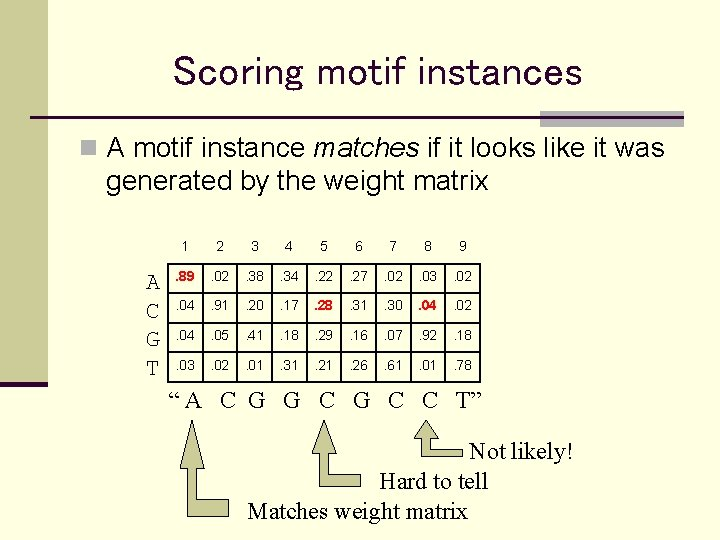Scoring motif instances n A motif instance matches if it looks like it was generated by the weight matrix A C G T 1 2 3 4 5 6 7 8 9 . 89 . 02 . 38 . 34 . 22 . 27 . 02 . 03 . 02 . 04 . 91 . 20 . 17 . 28 . 31 . 30 . 04 . 02 . 04 . 05 . 41 . 18 . 29 . 16 . 07 . 92 . 18 . 03 . 02 . 01 . 31 . 26 . 61 . 01 . 78 “ A C G G C C T” Not likely! Hard to tell Matches weight matrixLog likelihood ratio n A motif instance matches if it looks like it was generated by the weight matrix n Use log likelihood ratio i: the character at position i of the instance n Measures how much more like the weight matrix than like the background.Alternating approach Guess an initial weight matrix 2. Use weight matrix to predict instances in the input sequences 3. Use instances to predict a weight matrix 4. Repeat 2 & 3 until satisfied. 1. Examples: Gibbs sampler (Lawrence et al. ) MEME (expectation max. / Bailey, Elkan) ANN-Spec (neural net / Workman, Stormo)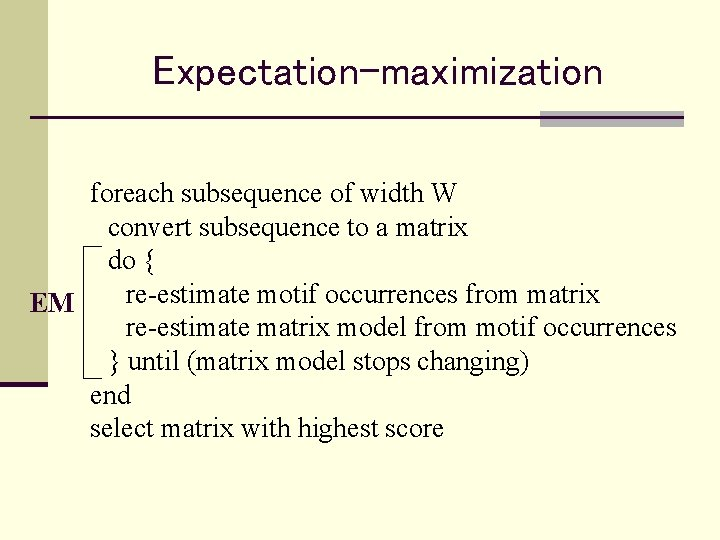Expectation-maximization foreach subsequence of width W convert subsequence to a matrix do { re-estimate motif occurrences from matrix EM re-estimate matrix model from motif occurrences } until (matrix model stops changing) end select matrix with highest score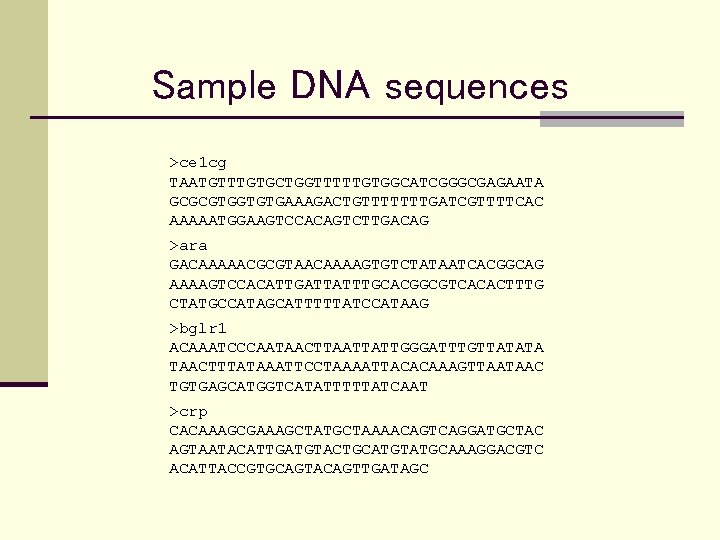Sample DNA sequences >ce 1 cg TAATGTTTGTGCTGGTTTTTGTGGCATCGGGCGAGAATA GCGCGTGGTGTGAAAGACTGTTTTTTTGATCGTTTTCAC AAAAATGGAAGTCCACAGTCTTGACAG >ara GACAAAAACGCGTAACAAAAGTGTCTATAATCACGGCAG AAAAGTCCACATTGATTATTTGCACGGCGTCACACTTTG CTATGCCATAGCATTTTTATCCATAAG >bglr 1 ACAAATCCCAATAACTTAATTATTGGGATTTGTTATATA TAACTTTATAAATTCCTAAAATTACACAAAGTTAATAAC TGTGAGCATGGTCATATTTTTATCAAT >crp CACAAAGCGAAAGCTATGCTAAAACAGTCAGGATGCTAC AGTAATACATTGATGTACTGCATGTATGCAAAGGACGTC ACATTACCGTGCAGTACAGTTGATAGCMotif occurrences >ce 1 cg taatgtttgtgctggtttttgtggcatcgggcgagaata gcgcgtggtgtgaaagactgtttt. TTTGATCGTTTTCAC aaaaatggaagtccacagtcttgacag >ara gacaaaaacgcgtaacaaaagtgtctataatcacggcag aaaagtccacattgatta. TTTGCACGGCGTCACactttg ctatgccatagcatttttatccataag >bglr 1 acaaatcccaataacttaattattgggatttgttatata taactttataaattcctaaaattacacaaagttaataac TGTGAGCATGGTCATatttttatcaat >crp cacaaagcgaaagctatgctaaaacagtcaggatgctac agtaatacattgatgtactgcatgta. TGCAAAGGACGTC ACattaccgtgcagtacagttgatagc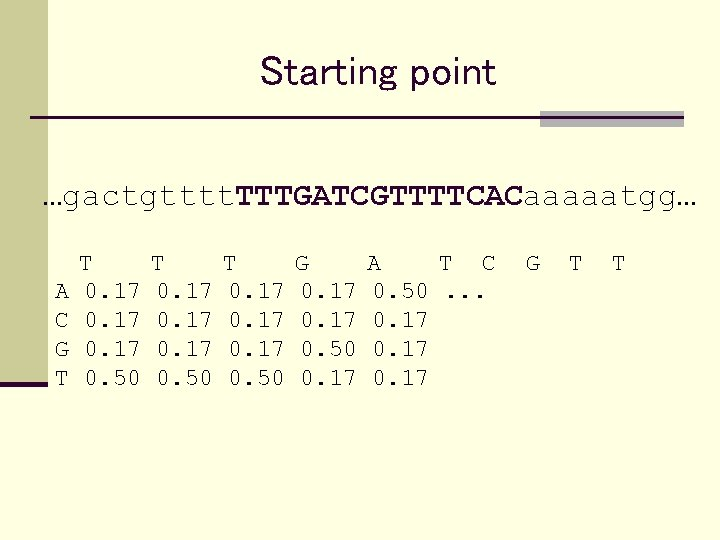Starting point …gactgtttt. TTTGATCGTTTTCACaaaaatgg… A C G T T 0. 17 0. 50 T 0. 17 0. 50 G 0. 17 0. 50 0. 17 A T C 0. 50. . . 0. 17 G T T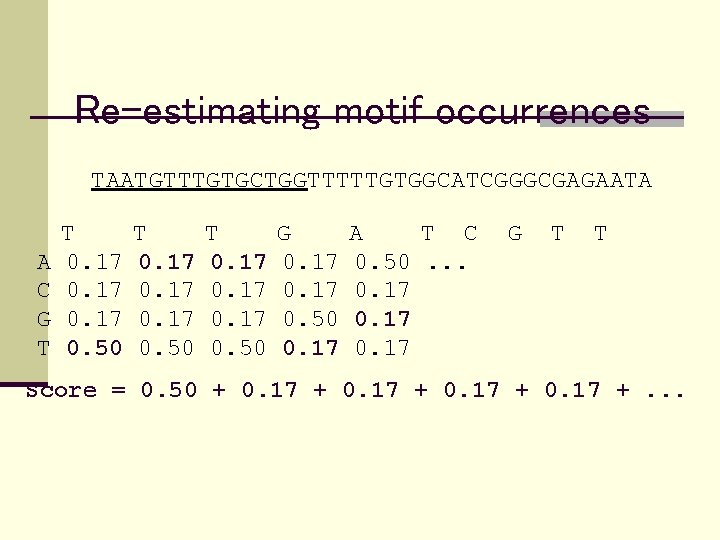Re-estimating motif occurrences TAATGTTTGTGCTGGTTTTTGTGGCATCGGGCGAGAATA A C G T T 0. 17 0. 50 T 0. 17 0. 50 G 0. 17 0. 50 0. 17 A T C 0. 50. . . 0. 17 G T T Score = 0. 50 + 0. 17 +. . .Scoring each subsequence Sequence: TGTGCTGGTTTTTGTGGCATCGGGCGAGAATA Subsequences Score TGTGCTGGTTTTTGT 2. 95 GTGCTGGTTTTTGTG 4. 62 TGCTGGTTTTTGTGG 2. 31 GCTGGTTTTTGTGGC. . . Select from each sequence the subsequence with maximal score.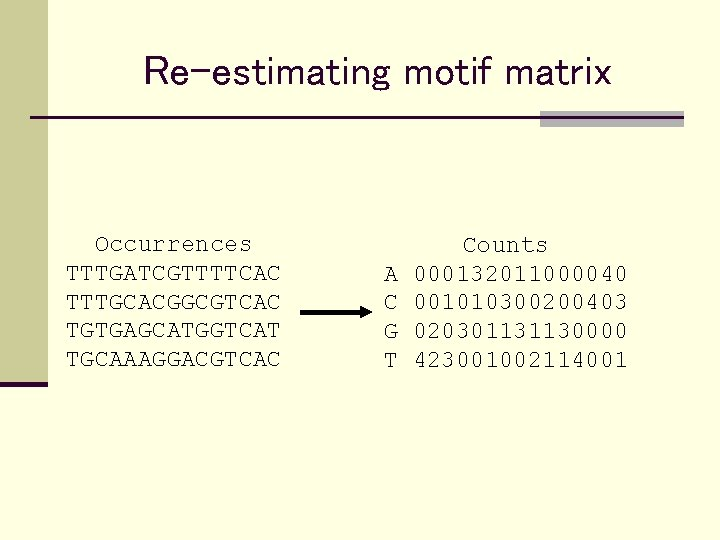Re-estimating motif matrix Occurrences TTTGATCGTTTTCAC TTTGCACGGCGTCAC TGTGAGCATGGTCAT TGCAAAGGACGTCAC A C G T Counts 000132011000040 001010300200403 020301131130000 423001002114001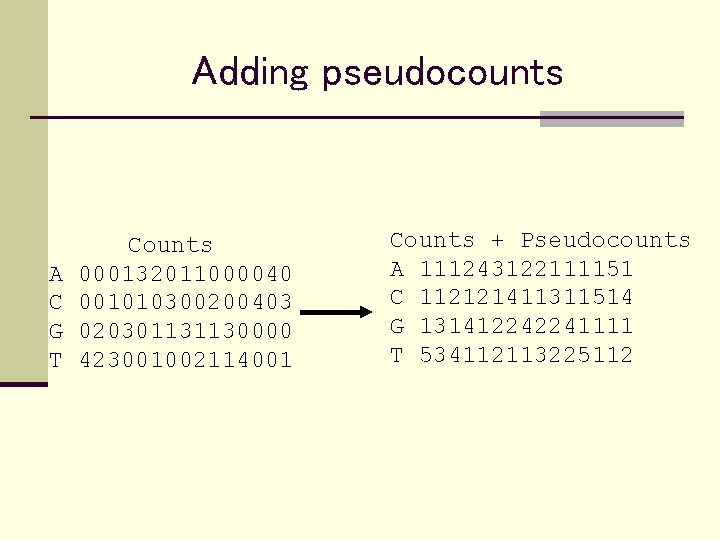Adding pseudocounts A C G T Counts 000132011000040 001010300200403 020301131130000 423001002114001 Counts + Pseudocounts A 111243122111151 C 112121411311514 G 131412242241111 T 534112113225112Converting to frequencies Counts + Pseudocounts A 111243122111151 C 112121411311514 G 131412242241111 T 534112113225112 A C G T T 0. 13 0. 63 T 0. 13 0. 38 T 0. 13 0. 25 0. 13 0. 50 G 0. 25 0. 13 0. 50 0. 13 A T C 0. 50. . . 0. 25 0. 13 G T T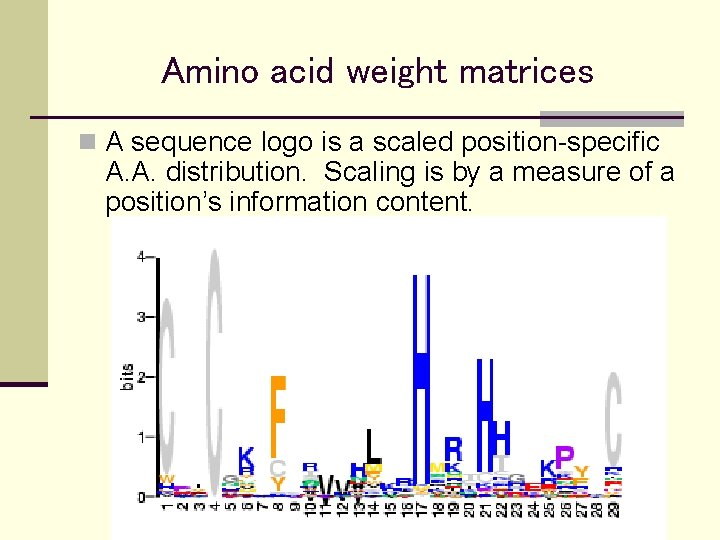Amino acid weight matrices n A sequence logo is a scaled position-specific A. A. distribution. Scaling is by a measure of a position’s information content.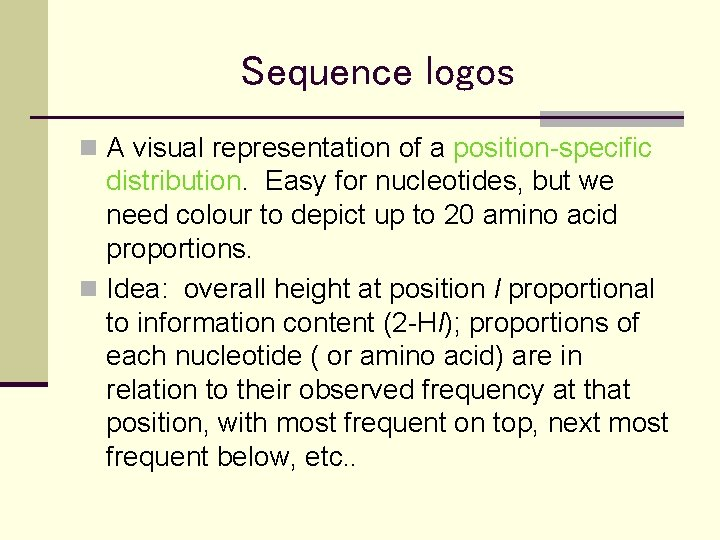Sequence logos n A visual representation of a position-specific distribution. Easy for nucleotides, but we need colour to depict up to 20 amino acid proportions. n Idea: overall height at position l proportional to information content (2 -Hl); proportions of each nucleotide ( or amino acid) are in relation to their observed frequency at that position, with most frequent on top, next most frequent below, etc. .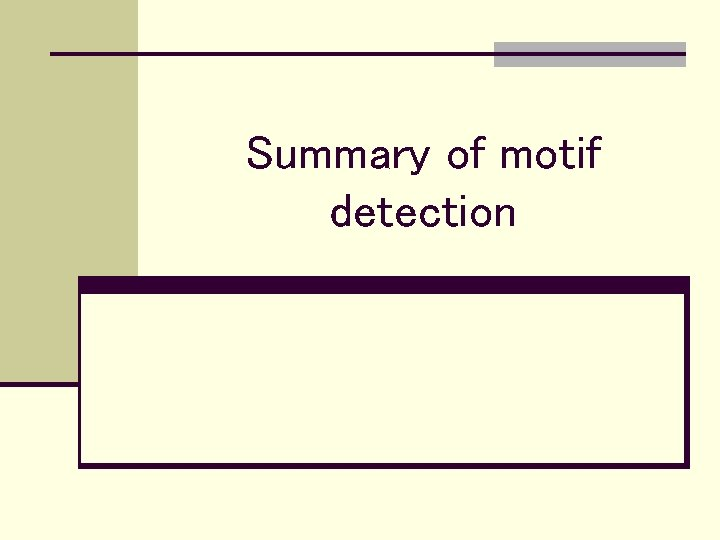Summary of motif detection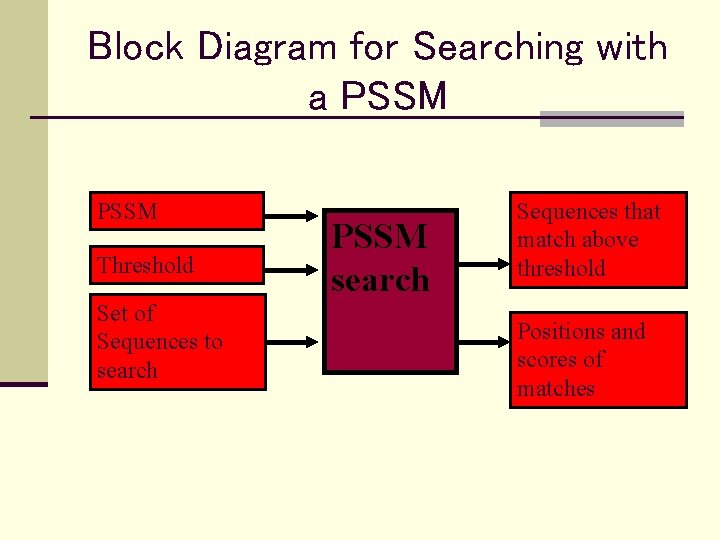Block Diagram for Searching with a PSSM Threshold Set of Sequences to search PSSM search Sequences that match above threshold Positions and scores of matchesBlock Diagram for Searching for sequences related to a family with a PSSM Set of Aligned Sequence Features Expected frequencies of each sequence element PSSM builder PSSM Threshold Set of Sequences to search PSSM search Sequences that match above threshold Positions and scores of matches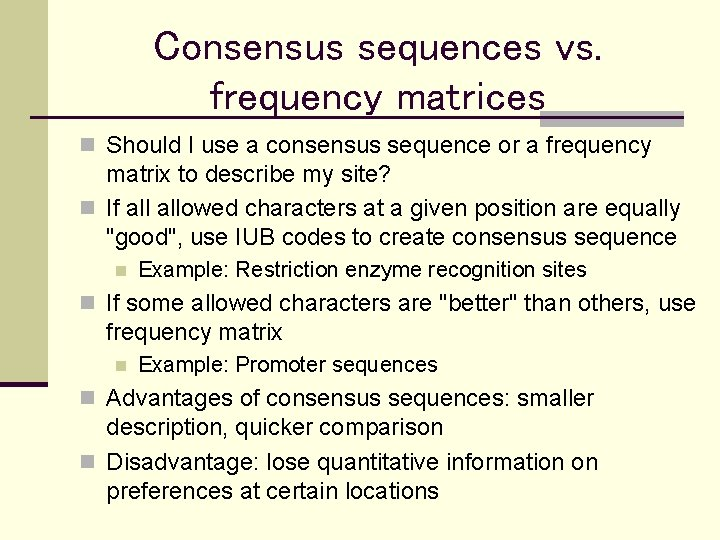Consensus sequences vs. frequency matrices n Should I use a consensus sequence or a frequency matrix to describe my site? n If allowed characters at a given position are equally "good", use IUB codes to create consensus sequence n Example: Restriction enzyme recognition sites n If some allowed characters are "better" than others, use frequency matrix n Example: Promoter sequences n Advantages of consensus sequences: smaller description, quicker comparison n Disadvantage: lose quantitative information on preferences at certain locations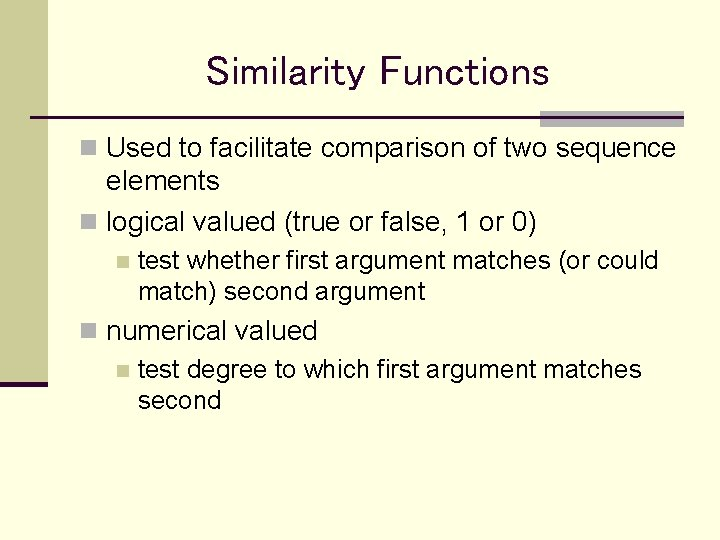Similarity Functions n Used to facilitate comparison of two sequence elements n logical valued (true or false, 1 or 0) n test whether first argument matches (or could match) second argument n numerical valued n test degree to which first argument matches second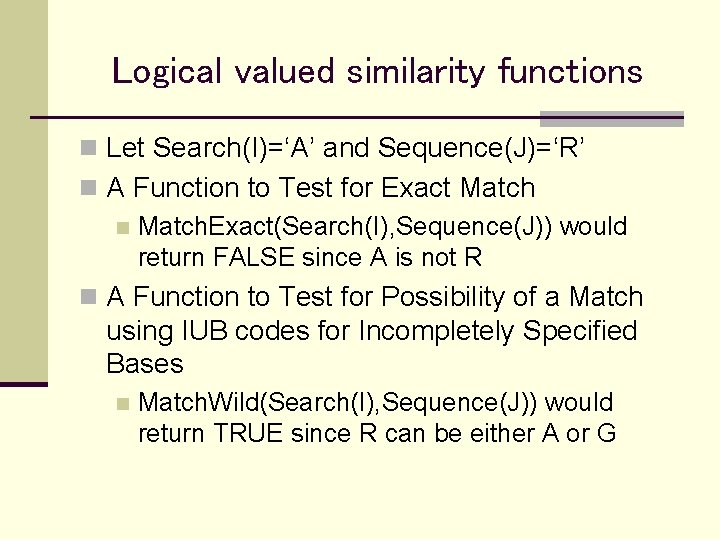Logical valued similarity functions n Let Search(I)=‘A’ and Sequence(J)=‘R’ n A Function to Test for Exact Match n Match. Exact(Search(I), Sequence(J)) would return FALSE since A is not R n A Function to Test for Possibility of a Match using IUB codes for Incompletely Specified Bases n Match. Wild(Search(I), Sequence(J)) would return TRUE since R can be either A or GNumerical valued similarity functions n return value could be probability (for DNA) n n Let Search(I) = 'A' and Sequence(J) = 'R' Similar. Nuc (Search(I), Sequence(J)) could return 0. 5 n since chances are 1 out of 2 that a purine is adenine n return value could be similarity (for protein) n n Let Seq 1(I) = 'K' (lysine) and Seq 2(J) = 'R' (arginine) Similar. Prot(Seq 1(I), Seq 2(J)) could return 0. 8 n since lysine is similar to arginine n usually use integer values for efficiency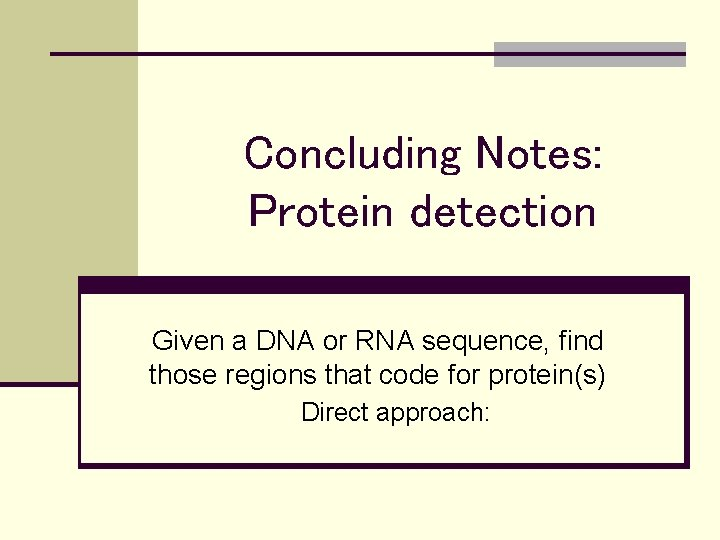Concluding Notes: Protein detection Given a DNA or RNA sequence, find those regions that code for protein(s) Direct approach: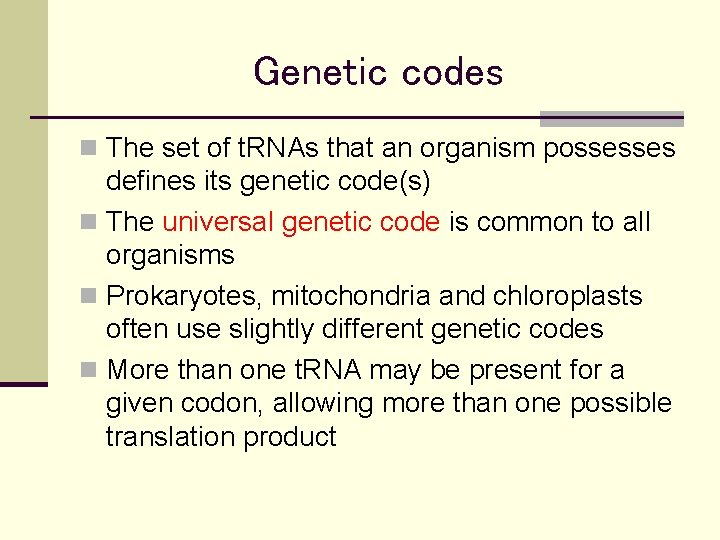Genetic codes n The set of t. RNAs that an organism possesses defines its genetic code(s) n The universal genetic code is common to all organisms n Prokaryotes, mitochondria and chloroplasts often use slightly different genetic codes n More than one t. RNA may be present for a given codon, allowing more than one possible translation product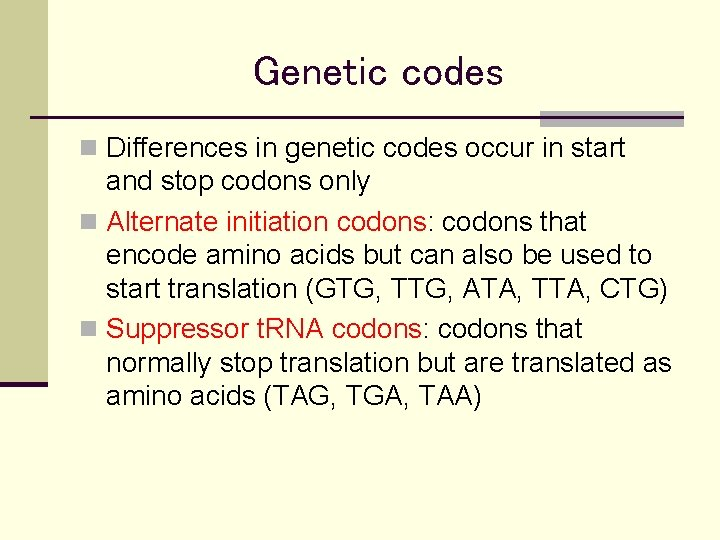Genetic codes n Differences in genetic codes occur in start and stop codons only n Alternate initiation codons: codons that encode amino acids but can also be used to start translation (GTG, TTG, ATA, TTA, CTG) n Suppressor t. RNA codons: codons that normally stop translation but are translated as amino acids (TAG, TGA, TAA)Reading Frames n Since nucleotide sequences are “read” three bases at a time, there are three possible “frames” in which a given nucleotide sequence can be “read” (in the forward direction) n Taking the complement of the sequence and reading in the reverse direction gives three more reading frames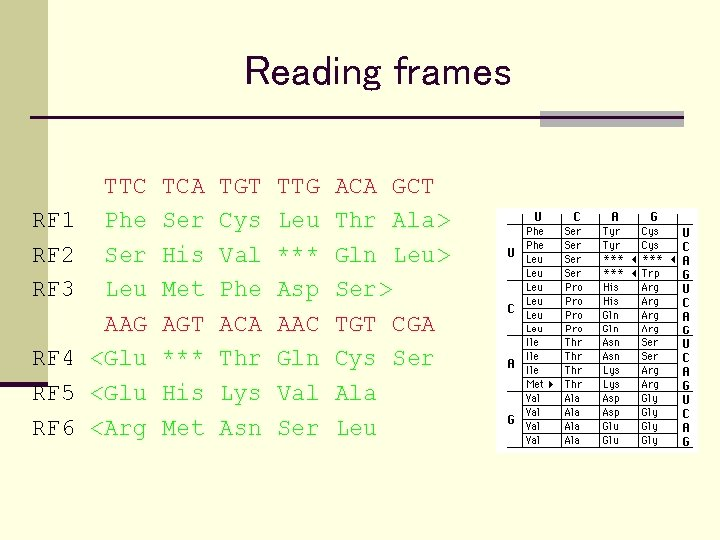Reading frames RF 1 RF 2 RF 3 RF 4 RF 5 RF 6 TTC Phe Ser Leu AAG <Glu <Arg TCA Ser His Met AGT *** His Met TGT Cys Val Phe ACA Thr Lys Asn TTG Leu *** Asp AAC Gln Val Ser ACA GCT Thr Ala> Gln Leu> Ser> TGT CGA Cys Ser Ala Leu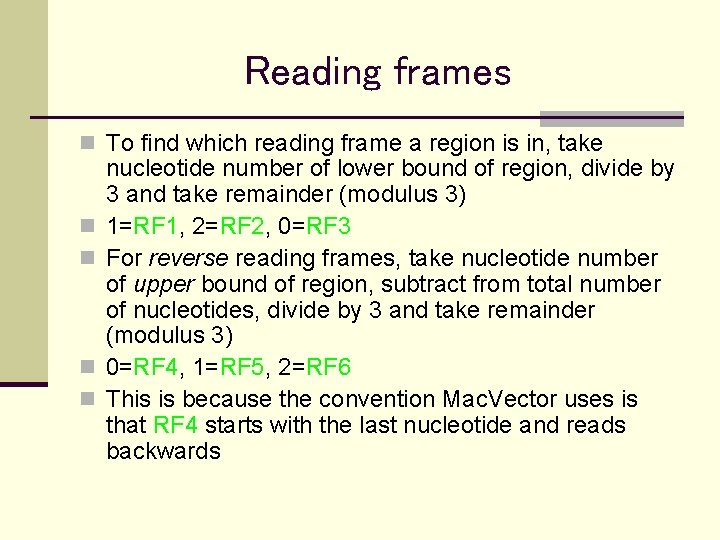Reading frames n To find which reading frame a region is in, take n n nucleotide number of lower bound of region, divide by 3 and take remainder (modulus 3) 1=RF 1, 2=RF 2, 0=RF 3 For reverse reading frames, take nucleotide number of upper bound of region, subtract from total number of nucleotides, divide by 3 and take remainder (modulus 3) 0=RF 4, 1=RF 5, 2=RF 6 This is because the convention Mac. Vector uses is that RF 4 starts with the last nucleotide and reads backwards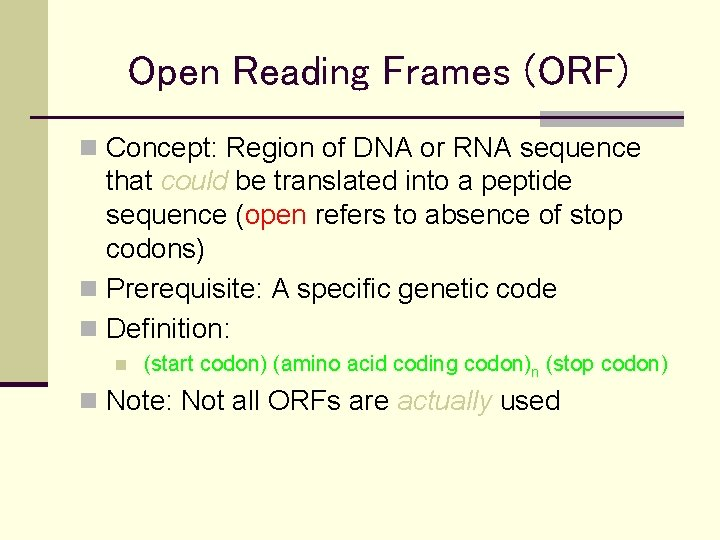Open Reading Frames (ORF) n Concept: Region of DNA or RNA sequence that could be translated into a peptide sequence (open refers to absence of stop codons) n Prerequisite: A specific genetic code n Definition: n (start codon) (amino acid coding codon)n (stop codon) n Note: Not all ORFs are actually usedBlock Diagram for Direct Search for ORFs Genetic code Both strands? Ends start/stop? Sequence to be searched Search Engine List of ORF positions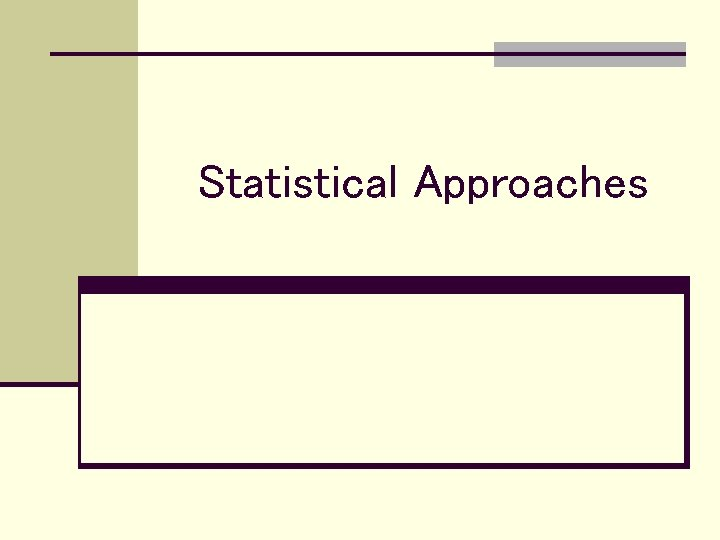Statistical Approaches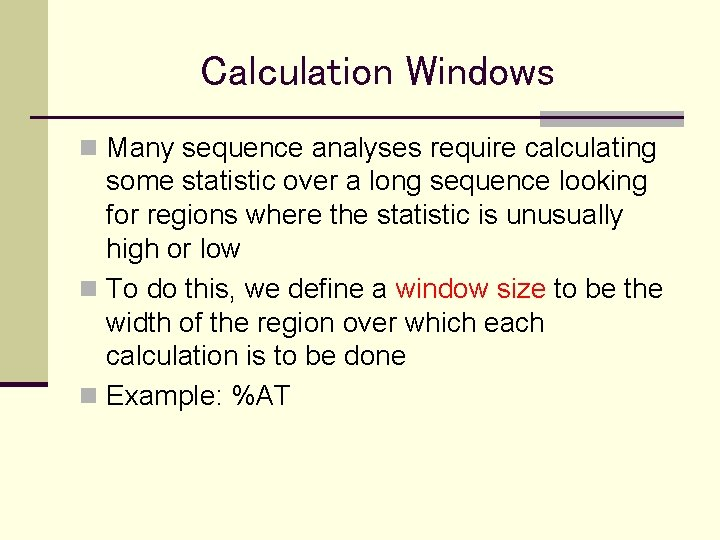Calculation Windows n Many sequence analyses require calculating some statistic over a long sequence looking for regions where the statistic is unusually high or low n To do this, we define a window size to be the width of the region over which each calculation is to be done n Example: %AT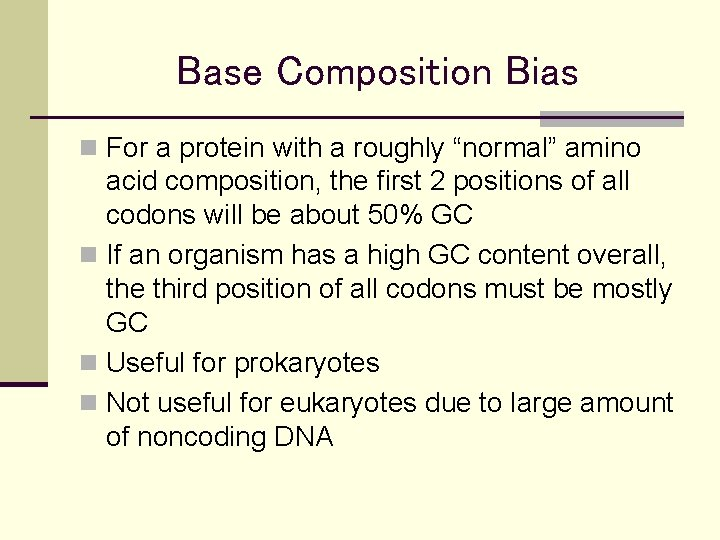Base Composition Bias n For a protein with a roughly “normal” amino acid composition, the first 2 positions of all codons will be about 50% GC n If an organism has a high GC content overall, the third position of all codons must be mostly GC n Useful for prokaryotes n Not useful for eukaryotes due to large amount of noncoding DNA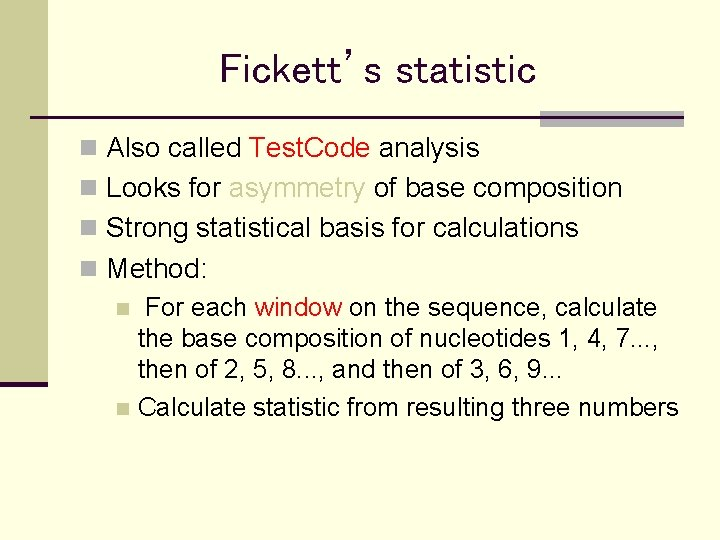Fickett’s statistic n Also called Test. Code analysis n Looks for asymmetry of base composition n Strong statistical basis for calculations n Method: n For each window on the sequence, calculate the base composition of nucleotides 1, 4, 7. . . , then of 2, 5, 8. . . , and then of 3, 6, 9. . . n Calculate statistic from resulting three numbers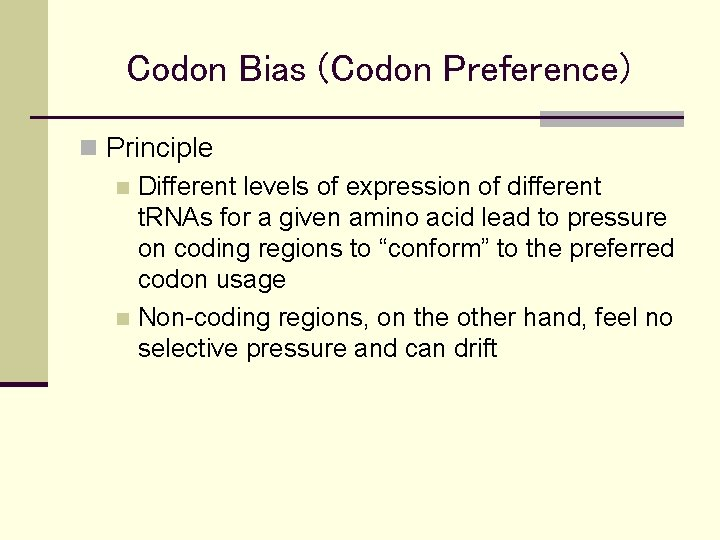Codon Bias (Codon Preference) n Principle n Different levels of expression of different t. RNAs for a given amino acid lead to pressure on coding regions to “conform” to the preferred codon usage n Non-coding regions, on the other hand, feel no selective pressure and can drift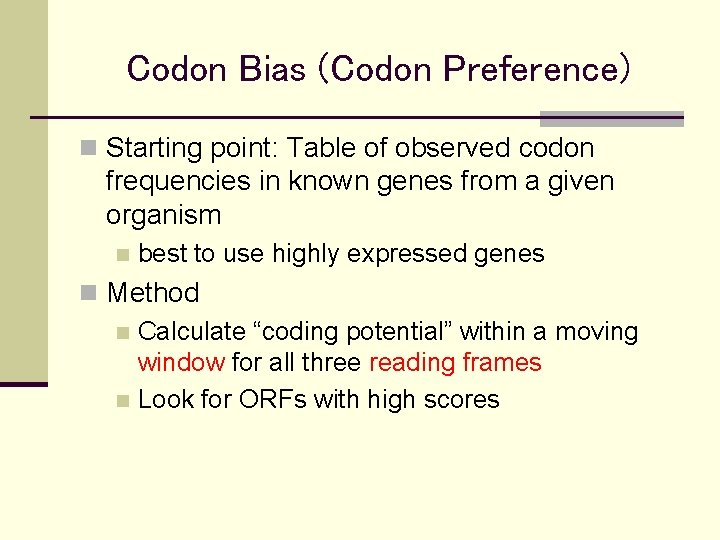Codon Bias (Codon Preference) n Starting point: Table of observed codon frequencies in known genes from a given organism n best to use highly expressed genes n Method n Calculate “coding potential” within a moving window for all three reading frames n Look for ORFs with high scoresCodon Bias (Codon Preference) n Works best for prokaryotes or unicellular eukaryotes because for multicellular eukaryotes, different pools of t. RNA may be expressed at different stages of development in different tissues n may have to group genes into sets n Codon bias can also be used to estimate protein expression levelPortion of D. melanogaster codon frequency table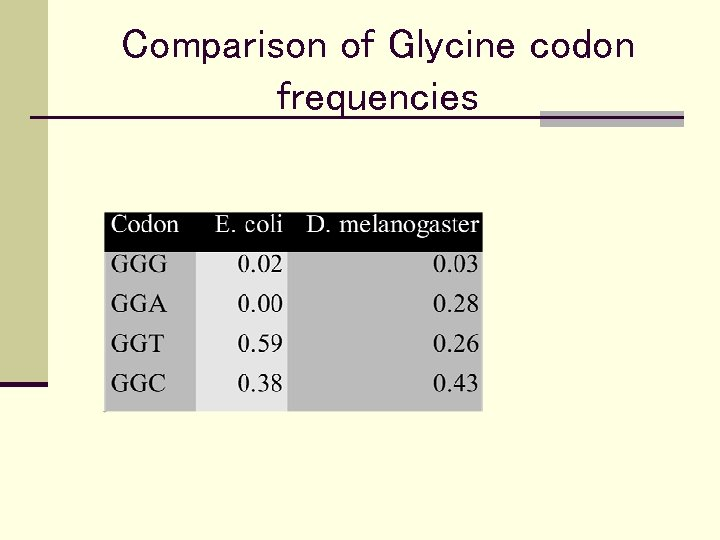Comparison of Glycine codon frequencies